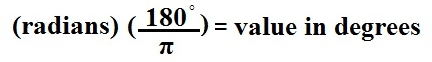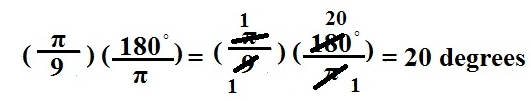﻿ How to Convert Radians to Degrees

# How to Convert from Radians to Degrees

The formula for converting from radians to degrees:You just put in the radian value that you want converted into degrees in the formula where it says (radians) and compute the equation and it will yield the value in degrees.

An example of this is below. We convert π/9 radians into its corresponding degree value:Related Resources